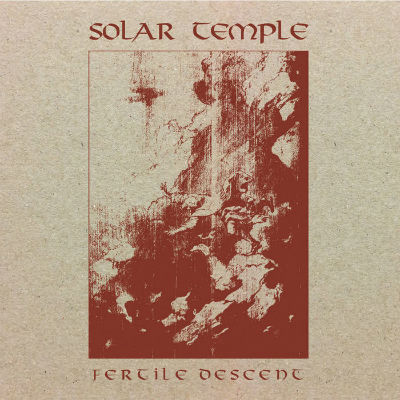## VM-UNDERGROUND

### Extreme Metal Fanzine est. 2012

Filter by: band
[%] - [[0-9]] - [A] - [B] - [C] - [D] - [E] - [F] - [G] - [H] - [I] - [J] - [K] - [L] - [M] - [N] - [O] - [P] - [Q] - [R] - [S] - [T] - [U] - [V] - [W] - [X] - [Y] - [Z]
Filter by: label
[[0-9]] - [A] - [B] - [C] - [D] - [E] - [F] - [G] - [H] - [I] - [J] - [K] - [L] - [M] - [N] - [O] - [P] - [Q] - [R] - [S] - [T] - [U] - [V] - [W] - [X] - [Y] - [Z]
Filter by: style
[B] - [C] - [D] - [E] - [F] - [G] - [H] - [I] - [P] - [S] - [T] - [V]
Filter by: country
[A] - [B] - [C] - [D] - [E] - [F] - [G] - [I] - [L] - [M] - [N] - [P] - [R] - [S] - [T] - [U]
Filter by: vmu-author
[A] - [B] - [C] - [D] - [E] - [F] - [G] - [H] - [I] - [J] - [K] - [L] - [M] - [N] - [O] - [P] - [R] - [S] - [T] - [V] - [X] - [Y] - [Z]

# Solar Temple – Fertile Descent## Info

From the Netherlands comes a wandering, experimental adventure through Black Metal, and post….whatever…this one took a few listens to grow on me. It definitely suffers from toolongitis with it being two tracks…both clocking in at over 15 minutes.

The impression that there were several songs within each of the two tracks remained even after the album became more favoured. But that doesn’t detract from the amount of musicianship and composition these two tracks contain. If you like your metal a bit on the shoegazey side this is going to be a gem for you. (TravisN)